Stokes Theorem

We set up a random function to test Stokes theorem.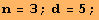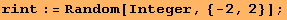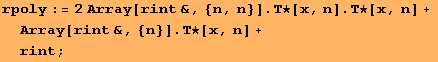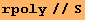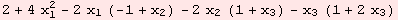We scale the operation ΤA(d ◦) by a factor of 2.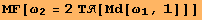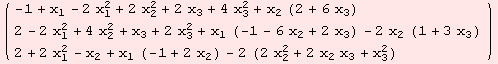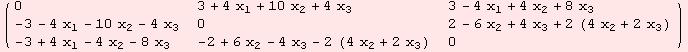The integrals in question are overand its boundary. The evaluations coincide.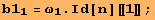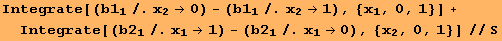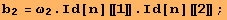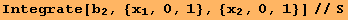The function to be integrated over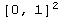is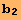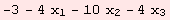The functions on the boundary ofare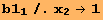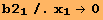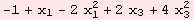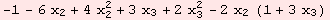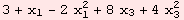Created by Mathematica  (December 22, 2006)